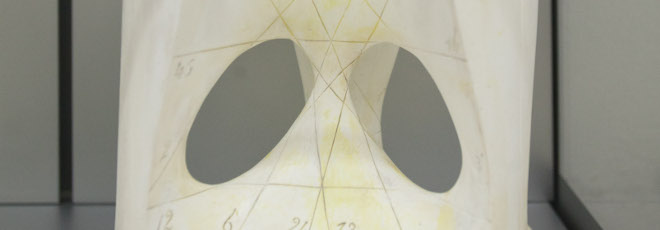## “On the volume of (half-)tubular neighborhoods of surfaces in sub-Riemannian geometry”

Wednesday 15 March 2023 h. 14:30 - Room 2BC30 - Tania Bossio (Padova, Dip. Mat.)

Abstract

In 1840 Steiner proved that the volume of the tubular neighborhood of a convex set in $\mathbb{R}^n$ is a polynomial of degree $n$ in the size of the tube. The coefficients of such a polynomial carry information about the curvature of the set. In this talk we present Steiner-like formulas in the framework of sub-Riemannian geometry. In particular, we introduce the three-dimensional sub-Riemaannian contact manifolds, which the first Heisenberg group is a special case of. Then, we show the asymptotic expansion of the volume of the half-tubular neighborhood of a surface and provide a geometric interpretation of the coefficients in terms of sub-Riemannian curvature objects.

Seminario Dottorato